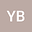•In this paper we give Navier-Stokes system associated with the Weinstein operator $(NSW)$ (see.\eqref{11}), We study the existence and uniqueness of solutions to equations (NSW) in \$L_{\alpha}^{p}\left(\mathbb{R}_{+}^{d+1}\right), 2 \alpha+d+2